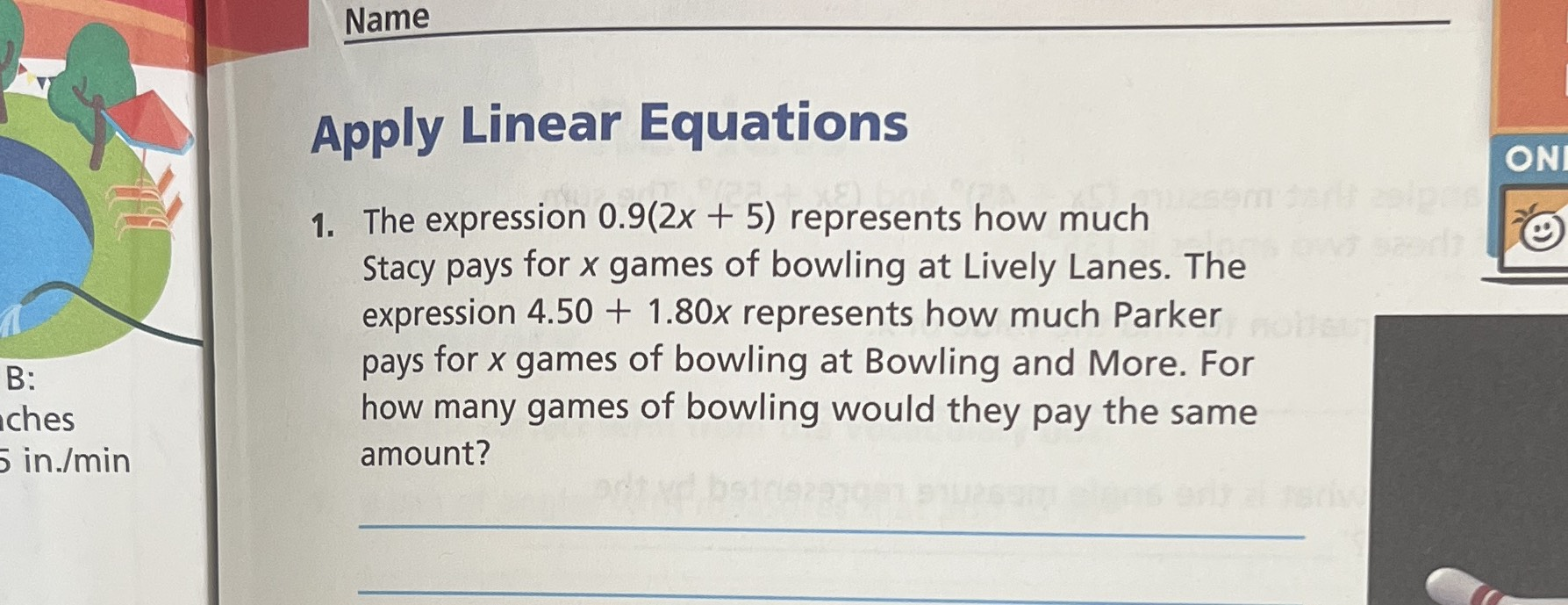### ¿Todavía tienes preguntas de matemáticas?

Pregunte a nuestros tutores expertos
Algebra
Pregunta1. The expression $$0.9 ( 2 x + 5 )$$ represents how much Stacy pays for $$x$$ games of bowling at Lively Lanes. The expression $$4.50 + 1.80 x$$ represents how much Parker pays for $$x$$ games of bowling at Bowling and More. For how many games of bowling would they pay the same amount?